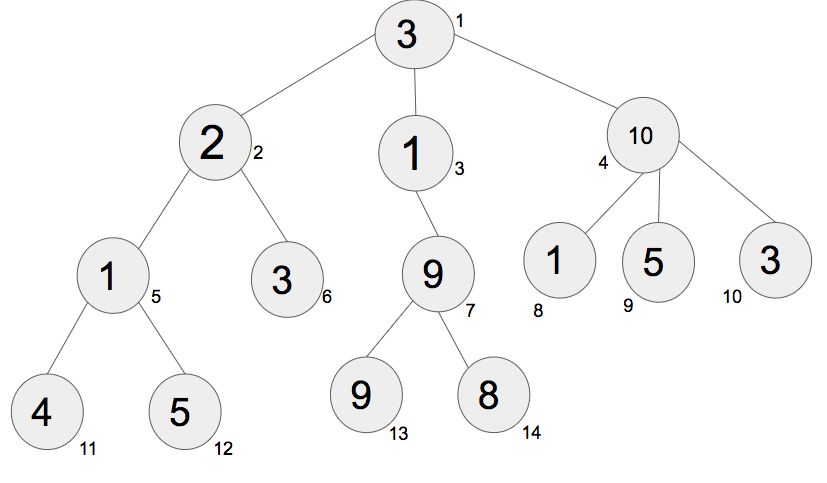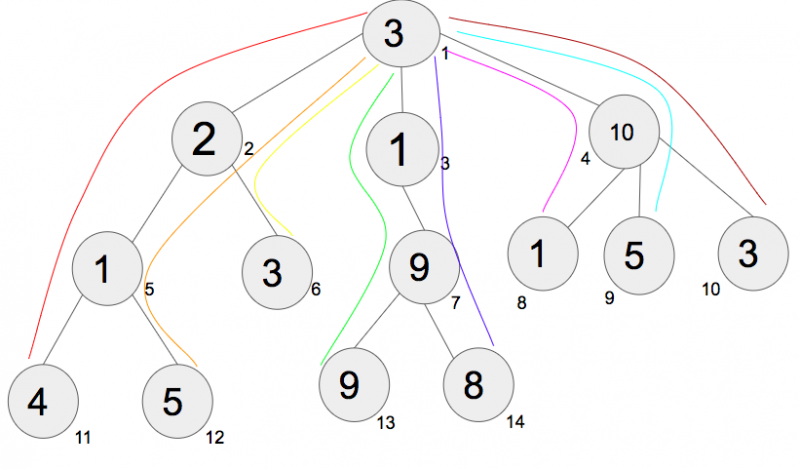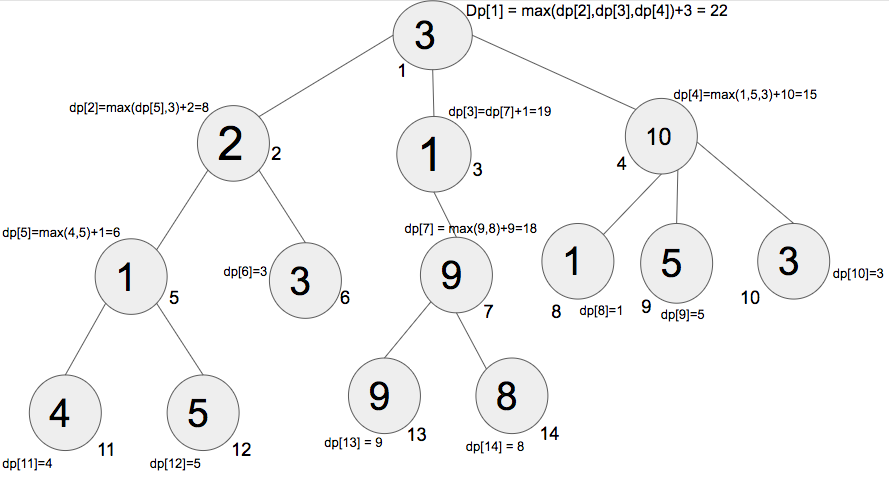# Dynamic Programming on Trees | Set-1

Dynamic Programming(DP) is a technique to solve problems by breaking them down into overlapping sub-problems which follows the optimal substructure. There are various problems using DP like subset sum, knapsack, coin change etc. DP can also be applied on trees to solve some specific problems.
Pre-requisite: DFS
Given a tree with N nodes and N-1 edges, calculate the maximum sum of the node values from root to any of the leaves without re-visiting any node.Given above is a diagram of a tree with N=14 nodes and N-1=13 edges. The values at node being 3, 2, 1, 10, 1, 3, 9, 1, 5, 3, 4, 5, 9 and 8 respectively for nodes 1, 2, 3, 4….14.
The diagram below shows all the paths from root to leaves :All the paths are marked by different colors :
Path 1(red, 3-2-1-4) : sum of all node values = 10
Path 2(orange, 3-2-1-5) : sum of all node values = 11
Path 3(yellow, 3-2-3) : sum of all node values = 8
Path 4(green, 3-1-9-9) : sum of all node values = 22
Path 5(violet, 3-1-9-8) : sum of all node values = 21
Path 6(pink, 3-10-1) : sum of all node values = 14
Path 7(blue, 3-10-5) : sum of all node values = 18
Path 8(brown, 3-10-3) : sum of all node values = 16
The answer is 22, as Path 4 has the maximum sum of values of nodes in its path from a root to leaves.

The greedy approach fails in this case. Starting from the root and take 3 from the first level, 10 from the next level and 5 from the third level greedily. Result is path-7 if after following the greedy approach, hence do not apply greedy approach over here.
The problem can be solved using Dynamic Programming on trees. Start memoizing from the leaves and add the maximum of leaves to the root of every sub-tree. At the last step, there will be root and the sub-tree under it, adding the value at node and maximum of sub-tree will give us the maximum sum of the node values from root to any of the leaves.The diagram above shows how to start from the leaves and add the maximum of leaves of a sub-tree to its root. Move upward and repeat the same procedure of storing the maximum of every sub-tree leaves and adding it to its root. In this example, the maximum of node 11 and 12 is taken to count and then added to node 5 (In this sub-tree, 5 is the root and 11, 12 are its leaves). Similarly, the maximum of node 13 and 14 is taken to count and then added to node 7. Repeat the steps for every sub-tree till we reach the node.

Let DPi be the maximum summation of node values in the path between i and any of its leaves moving downwards. Traverse the tree using DFS traversal. Store the maximum of all the leaves of the sub-tree, and add it to the root of the sub-tree. At the end, DP1 will have the maximum sum of the node values from root to any of the leaves without re-visiting any node.
Below is the implementation of the above idea :

## C++

 `// C++ code to find the maximum path sum` `#include ` `using` `namespace` `std;`   `int` `dp;`   `// function for dfs traversal and to store the` `// maximum value in dp[] for every node till the leaves` `void` `dfs(``int` `a[], vector<``int``> v[], ``int` `u, ``int` `parent)` `{` `    ``// initially dp[u] is always a[u]` `    ``dp[u] = a[u - 1];`   `    ``// stores the maximum value from nodes` `    ``int` `maximum = 0;`   `    ``// traverse the tree` `    ``for` `(``int` `child : v[u]) {`   `        ``// if child is parent, then we continue` `        ``// without recursing further` `        ``if` `(child == parent)` `            ``continue``;`   `        ``// call dfs for further traversal` `        ``dfs(a, v, child, u);`   `        ``// store the maximum of previous visited node` `        ``// and present visited node` `        ``maximum = max(maximum, dp[child]);` `    ``}`   `    ``// add the maximum value returned to the parent node` `    ``dp[u] += maximum;` `}`   `// function that returns the maximum value` `int` `maximumValue(``int` `a[], vector<``int``> v[])` `{` `    ``dfs(a, v, 1, 0);` `    ``return` `dp;` `}`   `// Driver Code` `int` `main()` `{` `    ``// number of nodes` `    ``int` `n = 14;`   `    ``// adjacency list` `    ``vector<``int``> v[n + 1];`   `    ``// create undirected edges` `    ``// initialize the tree given in the diagram` `    ``v.push_back(2), v.push_back(1);` `    ``v.push_back(3), v.push_back(1);` `    ``v.push_back(4), v.push_back(1);` `    ``v.push_back(5), v.push_back(2);` `    ``v.push_back(6), v.push_back(2);` `    ``v.push_back(7), v.push_back(3);` `    ``v.push_back(8), v.push_back(4);` `    ``v.push_back(9), v.push_back(4);` `    ``v.push_back(10), v.push_back(4);` `    ``v.push_back(11), v.push_back(5);` `    ``v.push_back(12), v.push_back(5);` `    ``v.push_back(13), v.push_back(7);` `    ``v.push_back(14), v.push_back(7);`   `    ``// values of node 1, 2, 3....14` `    ``int` `a[] = { 3, 2, 1, 10, 1, 3, 9, 1, 5, 3, 4, 5, 9, 8 };`   `    ``// function call` `    ``cout << maximumValue(a, v);`   `    ``return` `0;` `}`

## Java

 `// Java code to find the maximum path sum ` `import` `java.util.Vector;`   `class` `GFG` `{` `    ``static` `int``[] dp = ``new` `int``[``100``];`   `    ``// function for dfs traversal and to ` `    ``// store the maximum value in dp[] ` `    ``// for every node till the leaves` `    ``static` `void` `dfs(``int``[] a, Vector[] v, ` `                    ``int` `u, ``int` `parent) ` `    ``{`   `        ``// initially dp[u] is always a[u]` `        ``dp[u] = a[u - ``1``];`   `        ``// stores the maximum value from nodes` `        ``int` `maximum = ``0``;`   `        ``// traverse the tree` `        ``for` `(``int` `child : v[u]) ` `        ``{`   `            ``// if child is parent, then we continue` `            ``// without recursing further` `            ``if` `(child == parent)` `                ``continue``;`   `            ``// call dfs for further traversal` `            ``dfs(a, v, child, u);`   `            ``// store the maximum of previous visited ` `            ``// node and present visited node` `            ``maximum = Math.max(maximum, dp[child]);` `        ``}`   `        ``// add the maximum value returned` `        ``// to the parent node` `        ``dp[u] += maximum;` `    ``}`   `    ``// function that returns the maximum value` `    ``static` `int` `maximumValue(``int``[] a, ` `                            ``Vector[] v)` `    ``{` `        ``dfs(a, v, ``1``, ``0``);` `        ``return` `dp[``1``];` `    ``}`   `    ``// Driver Code` `    ``public` `static` `void` `main(String[] args) ` `    ``{`   `        ``// Driver Code` `        ``int` `n = ``14``;`   `        ``// adjacency list` `        ``@SuppressWarnings``(``"unchecked"``)` `        ``Vector[] v = ``new` `Vector[n + ``1``];`   `        ``for` `(``int` `i = ``0``; i < v.length; i++)` `            ``v[i] = ``new` `Vector<>();`   `        ``// create undirected edges` `        ``// initialize the tree given in the diagram` `        ``v[``1``].add(``2``); v[``2``].add(``1``);` `        ``v[``1``].add(``3``); v[``3``].add(``1``);` `        ``v[``1``].add(``4``); v[``4``].add(``1``);` `        ``v[``2``].add(``5``); v[``5``].add(``2``);` `        ``v[``2``].add(``6``); v[``6``].add(``2``);` `        ``v[``3``].add(``7``); v[``7``].add(``3``);` `        ``v[``4``].add(``8``); v[``8``].add(``4``);` `        ``v[``4``].add(``9``); v[``9``].add(``4``);` `        ``v[``4``].add(``10``); v[``10``].add(``4``);` `        ``v[``5``].add(``11``); v[``11``].add(``5``);` `        ``v[``5``].add(``12``); v[``12``].add(``5``);` `        ``v[``7``].add(``13``); v[``13``].add(``7``);` `        ``v[``7``].add(``14``); v[``14``].add(``7``);`   `        ``// values of node 1, 2, 3....14` `        ``int` `a[] = { ``3``, ``2``, ``1``, ``10``, ``1``, ``3``, ``9``, ` `                    ``1``, ``5``, ``3``, ``4``, ``5``, ``9``, ``8` `};`   `        ``// function call` `        ``System.out.println(maximumValue(a, v));` `    ``}` `}`   `// This code is contributed by` `// sanjeev2552`

## Python3

 `# Python3 code to find the maximum path sum ` `dp ``=` `[``0``]``*``100`   `# Function for dfs traversal and` `# to store the maximum value in` `# dp[] for every node till the leaves ` `def` `dfs(a, v, u, parent):` `    `  `    ``# Initially dp[u] is always a[u]` `    ``dp[u] ``=` `a[u ``-` `1``]` `    `  `    ``# Stores the maximum value from nodes` `    ``maximum ``=` `0` `    `  `    ``# Traverse the tree` `    ``for` `child ``in` `v[u]:` `        `  `        ``# If child is parent, then we continue ` `        ``# without recursing further ` `        ``if` `child ``=``=` `parent:` `            ``continue` `        `  `        ``# Call dfs for further traversal ` `        ``dfs(a, v, child, u)` `        `  `        ``# Store the maximum of previous visited  ` `        ``# node and present visited node ` `        ``maximum ``=` `max``(maximum, dp[child])` `        `  `    ``# Add the maximum value ` `    ``# returned to the parent node ` `    ``dp[u] ``+``=` `maximum`     `# Function that returns the maximum value` `def` `maximumValue(a, v):` `    ``dfs(a, v, ``1``, ``0``)` `    ``return` `dp[``1``]`   `# Driver Code ` `def` `main():` `    `  `    ``# Number of nodes ` `    ``n ``=` `14` `    `  `    ``# Adjacency list as a dictionary` `    ``v ``=` `{}` `    ``for` `i ``in` `range``(n ``+` `1``):` `        ``v[i] ``=` `[]` `        `  `    ``# Create undirected edges ` `    ``# initialize the tree given in the diagram ` `    ``v[``1``].append(``2``), v[``2``].append(``1``)` `    ``v[``1``].append(``3``), v[``3``].append(``1``) ` `    ``v[``1``].append(``4``), v[``4``].append(``1``) ` `    ``v[``2``].append(``5``), v[``5``].append(``2``) ` `    ``v[``2``].append(``6``), v[``6``].append(``2``) ` `    ``v[``3``].append(``7``), v[``7``].append(``3``) ` `    ``v[``4``].append(``8``), v[``8``].append(``4``) ` `    ``v[``4``].append(``9``), v[``9``].append(``4``) ` `    ``v[``4``].append(``10``), v[``10``].append(``4``) ` `    ``v[``5``].append(``11``), v[``11``].append(``5``) ` `    ``v[``5``].append(``12``), v[``12``].append(``5``) ` `    ``v[``7``].append(``13``), v[``13``].append(``7``) ` `    ``v[``7``].append(``14``), v[``14``].append(``7``) ` `    `  `    ``# Values of node 1, 2, 3....14 ` `    ``a ``=` `[ ``3``, ``2``, ``1``, ``10``, ``1``, ``3``, ``9``,` `          ``1``, ``5``, ``3``, ``4``, ``5``, ``9``, ``8` `]` `    `  `    ``# Function call` `    ``print``(maximumValue(a, v))` `main()`   `# This code is contributed by stutipathak31jan  `

## C#

 `// C# code to find the maximum path sum ` `using` `System;` `using` `System.Collections.Generic;`   `class` `GFG` `{` `    ``static` `int``[] dp = ``new` `int``;`   `    ``// function for dfs traversal and to ` `    ``// store the maximum value in []dp ` `    ``// for every node till the leaves` `    ``static` `void` `dfs(``int``[] a, List<``int``>[] v, ` `                    ``int` `u, ``int` `parent) ` `    ``{`   `        ``// initially dp[u] is always a[u]` `        ``dp[u] = a[u - 1];`   `        ``// stores the maximum value from nodes` `        ``int` `maximum = 0;`   `        ``// traverse the tree` `        ``foreach``(``int` `child ``in` `v[u]) ` `        ``{`   `            ``// if child is parent, then we continue` `            ``// without recursing further` `            ``if` `(child == parent)` `                ``continue``;`   `            ``// call dfs for further traversal` `            ``dfs(a, v, child, u);`   `            ``// store the maximum of previous visited ` `            ``// node and present visited node` `            ``maximum = Math.Max(maximum, dp[child]);` `        ``}`   `        ``// add the maximum value returned` `        ``// to the parent node` `        ``dp[u] += maximum;` `    ``}`   `    ``// function that returns the maximum value` `    ``static` `int` `maximumValue(``int``[] a, ` `                            ``List<``int``>[] v)` `    ``{` `        ``dfs(a, v, 1, 0);` `        ``return` `dp;` `    ``}`   `    ``// Driver Code` `    ``public` `static` `void` `Main(String[] args) ` `    ``{`   `        ``// Driver Code` `        ``int` `n = 14;`   `        ``// adjacency list`   `        ``List<``int``>[] v = ``new` `List<``int``>[n + 1];`   `        ``for` `(``int` `i = 0; i < v.Length; i++)` `            ``v[i] = ``new` `List<``int``>();`   `        ``// create undirected edges` `        ``// initialize the tree given in the diagram` `        ``v.Add(2); v.Add(1);` `        ``v.Add(3); v.Add(1);` `        ``v.Add(4); v.Add(1);` `        ``v.Add(5); v.Add(2);` `        ``v.Add(6); v.Add(2);` `        ``v.Add(7); v.Add(3);` `        ``v.Add(8); v.Add(4);` `        ``v.Add(9); v.Add(4);` `        ``v.Add(10); v.Add(4);` `        ``v.Add(11); v.Add(5);` `        ``v.Add(12); v.Add(5);` `        ``v.Add(13); v.Add(7);` `        ``v.Add(14); v.Add(7);`   `        ``// values of node 1, 2, 3....14` `        ``int` `[]a = { 3, 2, 1, 10, 1, 3, 9, ` `                    ``1, 5, 3, 4, 5, 9, 8 };`   `        ``// function call` `        ``Console.WriteLine(maximumValue(a, v));` `    ``}` `}`   `// This code is contributed by PrinciRaj1992`

Output

`22`

Time Complexity: O(N), where N is the number of nodes.

Attention reader! Don’t stop learning now. Get hold of all the important DSA concepts with the DSA Self Paced Course at a student-friendly price and become industry ready.

My Personal Notes arrow_drop_upStriver(underscore)79 at Codechef and codeforces D

If you like GeeksforGeeks and would like to contribute, you can also write an article using contribute.geeksforgeeks.org or mail your article to contribute@geeksforgeeks.org. See your article appearing on the GeeksforGeeks main page and help other Geeks.

Please Improve this article if you find anything incorrect by clicking on the "Improve Article" button below.